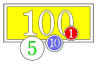# Rounding NumbersScientists have a convention for rounding numbers to the appropriate number of significant figures. In the age of calculators that mindlessly present many digits more than are significant, knowing how to round numbers "to the nearest tenth" or "to the nearest millionth" is essential.

## Teaching Tips

When students are asked to calculate numbers to a certain number of significant figures they almost invariably have to know how to properly round off the answer they get with their calculator. While most students think they know how to round numbers, there are two common mistakes that are made.

• First, some students believe that you simply drop any digits to the right of the last significant figure (thus 'cutting off' the number rather than rounding it off).
• Second, when the digit to the immediate right of the last significant figure is 5 many students simply drop the 5 and add 1 to the last significant figure (the automatically round up). This creates an inherent bias in rounding numbers up more frequently than rounding numbers down.

A discussion of the rules of rounding should occur before students are asked to calculate and report their results for any exercise.

## Teaching Resources

For a short discussion of rounding numbers that includes specific examples you can download this file. You might want to give this to your students as a quick primer or use it to create a problem set for your students. (Written by Vince Cronin, Baylor University.)

Primer on Rounding Numbers (Microsoft Word PRIVATE FILE 30kB Apr2 14)